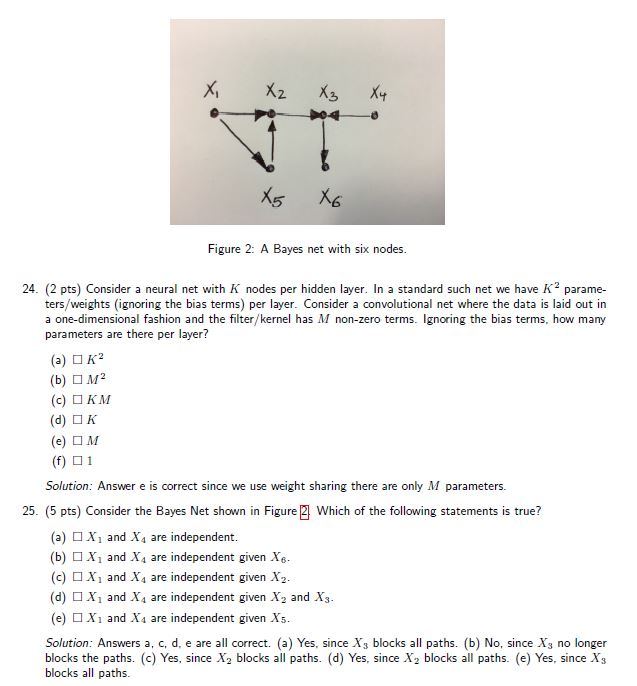### 2016 - Q25

Hello,I don't really get how to determine the independencies...

Could somehow give me an explanation ?

Top comment

What do you mean by: "It seems that the solution a, b, c, d, e are not aligned correctly with the answers." ? Are those answers incorrect ?

Sorry, I was too quick with my reply. I didn't see the directed edges in the graph. The answers are correct. Graphical models are not part of the curriculum of this course anymore, but if you are interested, you can learn more from last year's handout. They contain a simple set of rules to determine conditional independence.

• ~It seems that the solution a, b, c, d, e are not aligned correctly with the answers.~

• In general, if you have variables x, y, z connected as x -- y -- z, the variables x and z are not independent. Intuitively, if you know the value of one, you may get some knowledge about the values that the other might take. The value of x tells you something about y, and your new knowledge about y tells you something about z.

If you already know the value of 'y', however ("given y"), then learning the value of x will not tell you anthing 'more' about z. So (z and x are independent given y).

Hope this helps!

What do you mean by: "It seems that the solution a, b, c, d, e are not aligned correctly with the answers." ? Are those answers incorrect ?

Is X1, X4, given X3 independent as well ?

Top comment

What do you mean by: "It seems that the solution a, b, c, d, e are not aligned correctly with the answers." ? Are those answers incorrect ?

Sorry, I was too quick with my reply. I didn't see the directed edges in the graph. The answers are correct. Graphical models are not part of the curriculum of this course anymore, but if you are interested, you can learn more from last year's handout. They contain a simple set of rules to determine conditional independence.